Maths-
General
Easy

Question

#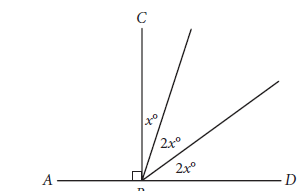In the figure above, point B lies on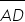. What is the value of 3x?

## 18365472Hint:

## The correct answer is: 54

### Explanation: We have given different parts of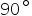angle in terms of x . We have to find the value of x . We know that the sum of all these angles will be. So, Using this we can easily find the value of x. Step 1 of 1:We have three parts ofangle as x,2x,2xNow, we know that the sum of these angles will be.so,Now the value of 3x will be =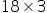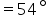#### With Turito Foundation.#### Get an Expert Advice From Turito.